# Chapter-4 Basic Geometrical Ideas Worksheet-1 for class 6 Maths

## Introduction

Geometry is about the properties and their structures which can be further categorize into:

• Plane Geometry: Plane geometry is about flat shapes such as lines, circles and triangles ... shapes can be drawn on paper.
• Solid geometry: Solid geometry is about three-dimensional objects such as cubes, prisms, cylinders, and spheres.

### Points

The point is a mark of position. A dot represents a point. We denote the point with the letters A, B, P, Q etc. The point has no length, breadth or thickness.

### Incidence Axioms on Lines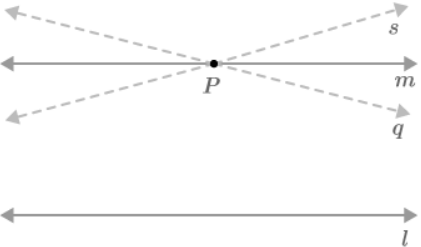• The line contains an infinite number of points
• An infinite number of lines can be drawn to pass a certain point
• One line can be drawn to pass the two points provided for point A and B.

### Collinear Points

Three or more points are called collinear points, if there is a line that contains them all.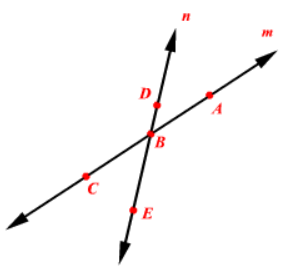### Intersecting Lines

Two lines with the same point are called intersecting lines. A common point in two given lines is called their meeting point. In the illustration, lines AB and CD meet at O.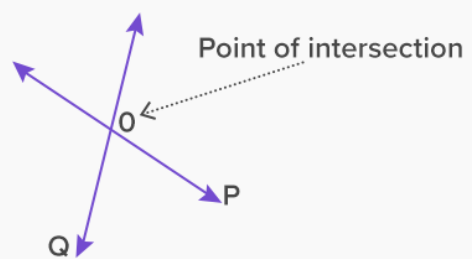### Parallel Lines

The two lines l and m in the plane are said to be parallel, if they do not have the same point and are labeled as l || m. The distance between two parallel lines remains the same.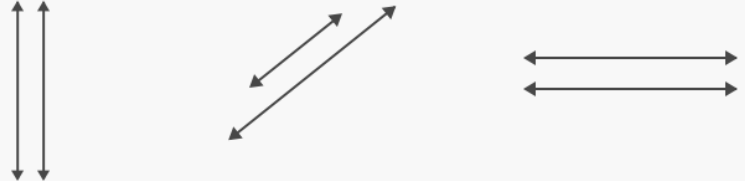### Polygons

Simple closed curves made up of line segments are called polygons. The line segments that form a polygon are the sides of a polygon. Any two sides with a common end point are called adjacent sides. The point of cross-cutting is called the vertex. The end points on the same side of the vertices are adjacent. The part of the line that connects any two adjacent vertices to the polygon is diagonal. The ABCDEF is a polygon.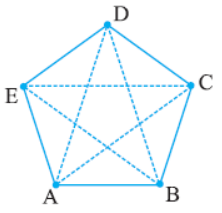The line sections AB, BC, CD, DE, DF, and FA are known as polygon ABCDEF components.

Any two sides with similar ends are called adjacent sides. AB and BC are adjacent sides with a common endpoint B.

The meeting place of the two sides of the polygon is known as the vertex. In the ABCDEF polygon, the sides of AB and BC meet in point B. Thus, point B is called the vertex of the polygon. Similarly, some vertices are A, C, D, E, and F.

A line that connects any two adjacent vertices of a polygon is known as its diagonal. In the next polygon ABCDEF, the diagonals are AC, AD, AE, BD, BE, BF, CE, CF, and DF.

### Solved Examples

Q1. A set of points with one end point and can be extended in one direction is

1. Line segment
2. Line
3. Ray
4. None

Ans. Correct answer is 1

A ray has only one end point.

Q2. Number of end points a line segment has

1. Three
2. None
3. Two
4. One

Ans. Correct answer is 3

Line segment is a part of line having two end points.

Q3. Number of lines that can be drawn from two distinct points on a plane

1. Two
2. One
3. Zero
4. Infinite

Ans. Correct answer is 2

We can draw only one line from two points.

Q4. PQ = 3 cm; RS = 6.2 cm. Then the measure of line segment whose length is equal to sum of PQ and RS is

1. 3.2 cm
2. 4 cm
3. 4.2 cm
4. 9.2 cm

Ans. Correct answer is 4

Clearly sum = 3 + 6.2 = 9.2 cm

Q5. How many lines can be drawn that pass through two different points?

1. 1
2. 2
3. 3
4. Infinite

Ans. Correct answer is 1

Only one line can be drawn which can pass through two different points.

Q6. Which of the following statement is true?

1. Every ray has a definite length
2. Every line has a definite length
3. Every line segment has a definite length
4. None the these.

Ans. Correct answer is 3

Every line segment has a definite length

Q7. The common end point of an angle is called

1. Vertex
2. Zero
3. End point
4. None of these

Ans. Correct answer is 1

Common end point of an angle is called vertex.

Q8. The interior and boundary of a triangle is called

1. Exterior
2. Interior
3. Triangular region
4. Plane

Ans. Correct answer is 3

Triangular region

Q9. Number of arcs made by a chord on a circle

1. 3
2. 2
3. 1
4. None

Ans. Correct answer is 2

Any part of a circle is called an arc, and a chord is a line segment whose end points always lie on the plane of the circle.

Q10. Each equal part of the circle is called

1. Semicircle
2. Major circle
3. Minor circle
4. None

Ans. Correct option is 1

Each half part of the circle is called semicircle.

Academic team of Physics Wallah prepared notes for CBSE Class 6 Basic of Geometry with important topic-wise questions. Students must practice the NCERT Class 6 Basic Geometrical Ideas worksheets and exercises with solutions that will help them revise essential concepts Class 6 Basic Geometrical Ideas. Academic team of Physics Wallah prepared short notes and all important Maths Formulas and Maths Symbols.

### Also Check:

Download Basic Geometrical Ideas Worksheet PDF given below